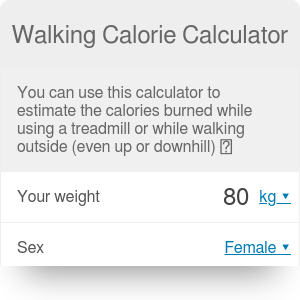lb
Slope
0%
Distance
km
Walking time
hrs
Walking speed
mph
Calories
kcal
Weight lost
lb

# Walking Calorie Calculator

By Bogna Haponiuk

No more tedious calculations after returning from the gym - this walking calorie calculator will calculate the calories burned walking or running on a treadmill. All that it needs are some basic information about your walking or running exercise, such as the distance and average speed, and it will provide you with the most accurate measure of how many calories does walking burn.

You can use this calculator in two ways: either to estimate the treadmill calorie loss or the calories burnt walking (for example, when you walk your dog). As the calculator takes the surface inclination into account, you can be sure that the result will be as precise as possible.

Make sure to take a look at our BMI calculator and the body fat calculator, too!

## How many calories does walking burn?

Each time you perform any exercise, such as walking or running, you burn some calories. The amount depends on a few factors: your speed, the time of exercising, your weight and the slope. Intuitively, if the grade is negative (you're on a decline), you burn less calories than if it is positive. For extreme slopes, use our hiking calculator instead.

This walking calorie calculator uses equations based on data presented in the study "Energy Cost of Running," by R. Margaria, P. Cerretelli, P. Aghemo, and G. Sassi. Equations were derived by shapesense.com.

## How to use the treadmill calorie calculator?

1. Enter your current weight. Don't cheat! :)
2. Enter the slope of the walking surface in percents. If you were walking uphill, the slope will be positive. If you were walking downhill - negative.
3. Enter two of the following values: the distance of your walk, the time it took you or the average speed. The walking calorie calculator will automatically calculate the third on its own.
4. That's all the data you need - the treadmill calorie calculator will hind the amount of calories burnt walking for you, too!
5. The last value that the calculator finds is the weight you lose if you burn that many calories. It is simply the number of calories divided by 7700 (the result is in kilograms).
Bogna Haponiuk

## Get the widget!

Walking Calorie Calculator can be embedded on your website to enrich the content you wrote and make it easier for your visitors to understand your message.

It is free, awesome and will keep people coming back!